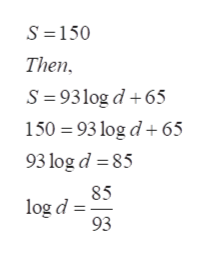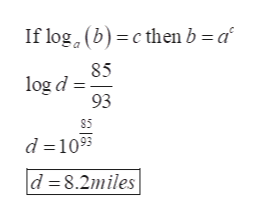# The wind speed near the center of a tornado is represented by the equation S=93logd+65, where d is the distance, in miles, that the tornado travels and S is the wind speed, in miles per hour.If the wind speed was 150 miles per hour, how many miles did the tornado travel? Round your answer to the nearest tenth.

Question
48 views

The wind speed near the center of a tornado is represented by the equation S=93logd+65, where d is the distance, in miles, that the tornado travels and S is the wind speed, in miles per hour.

If the wind speed was 150 miles per hour, how many miles did the tornado travel? Round your answer to the nearest tenth.

check_circle

star
star
star
star
star
1 Rating
Step 1

Refer to the question we need to find the distance, in miles, that the tornado travels when wind speed was 150 miles per hour. Also The wind speed near the center of a tornado is represented by the equation S=93logd+65, where d is the distance, in miles, that the tornado travels and S is the wind speed, in miles per hour.

Step 2

Now substitute the value of speed s=150 in the above equation and solve for d ,help_outlineImage TranscriptioncloseS 150 Then S 93log d65 150 93 log d65 93 log d 85 85 log d 93 fullscreen
Step 3

Now use the logarithm definition to s...help_outlineImage TranscriptioncloseIf log2 (b) cthen b a 85 log d 93 S5 d 109 d 8.2miles fullscreen

### Want to see the full answer?

See Solution

#### Want to see this answer and more?

Solutions are written by subject experts who are available 24/7. Questions are typically answered within 1 hour.*

See Solution
*Response times may vary by subject and question.
Tagged in

### Equations and In-equations